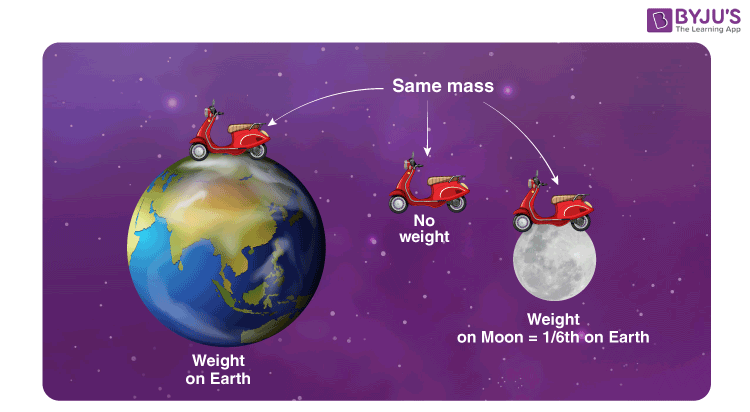# Measurement of Mass: Mass and Weight

Mass is a basic characteristic property of matter. It exists self-sufficiently and is independent of all other parameters such as the temperature, pressure, and the location of the object in space. Atomic mass is the mass of an atom expressed in atomic mass units. The matter has mass and occupies space. These two things are taught to us as soon as we can grasp these concepts. A matter is anything you can touch physically, so everything you see and interact with around you has a mass. Mass is often confused with another parameter. This confusion occurs due to the fact that this parameter is mistakenly used around the globe instead of mass due to its convenience and also due to the fact that we weigh things to find out their mass. This parameter is called weight. Let’s explore both of these essential parameters thoroughly.

## What is Mass?

Mass by definition refers to the amount of matter in a particular object. This value of the amount of matter i.e. mass of an object is an intrinsic value of that body and it can help us find out various other parameters that are dependent on the mass. Mass determines the strength of its mutual gravitational attraction to other bodies, its resistance to acceleration due to a force, Inertia, and mass can also be used to derive the energy content of a sample through the theory of Relativity using Albert Einstein’s E = mc2.

The SI Unit of mass is the kilogram (kg). A kilogram can be divided into 1000 grams and it was first defined as one cubic decimetre of water at the melting point of ice, i.e. 0o C. This was a very risky method of defining the unit of mass since the melting point of ice can be altered easily leading to changes in the value of a kilogram. Such changes in the basic units of science can cause a catastrophe which is why the Kilogram was redefined as the mass of the International Prototype Kilogram. International prototype Kilogram is a block of Platinum-Iridium alloy in the shape of a cylinder 39.17 millimetres in both height and diameter. Kilogram serves the function of everyday use perfectly well but it becomes unfeasible as a unit in the case of both extremely large and tiny masses.

## Atomic Mass Unit

For tiny and larger objects we use other units;

• Tonne(Metric Ton) is equal to 1000 kg
• The Atomic Mass Unit is used while dealing with atoms and molecules whose masses are so small that the kilogram becomes inconvenient. One atomic mass unit is defined as 1/12th the mass of a Carbon-12 atom. The value of 1 atomic mass unit is obtained as 1.66 x 10-27

### Measurement of mass

Measurement of mass is most commonly done by a Balance. The unknown mass of a body is compared with a known value of mass. We obtain the value of an unknown mass in terms of a known value of mass. A balance works in space and in places of no gravity as well since changes in gravity affect both the masses on the balance equally.## What is Weight?

Mass is not the same as weight. While mass is the intrinsic property of the body, weight is the measure of the force exerted on the mass of the body due to gravity. Mass refers to a universal value of the object whereas weight is a localized interpretation of the mass of the object. Weight is the effect of gravity and therefore we describe weight with the formula;

W = mg

Where m is the mass and g is the acceleration due to gravity at that particular location. The unit of measurement of weight is Force, the SI Unit of which is Newton. For example, an object that has a mass of 50 kg experiences a gravitational force i.e. weight which is equal to 50 x 9.8 = 490 Newton. So when you tell your friends you weight 50 kg you are telling them about your mass and not your weight. The same object albeit with the same mass of 50 kg will weigh 1/6th on the moon what it did on Earth. Weight and mass mean the same thing on Earth since the effects of gravity are fairly constant throughout the Earth. It was upon our venture into space that it became necessary to create a distinction between mass and weight.Here is a problem based on the weighing machine, the problem deals with an advanced question on how normal reaction and tension act in an accelerated pulley system when the support is provided by the man which is being measured.## Frequently Asked Questions – FAQs

Q1

### What is mass?

Mass is the measure of the quantity of matter in an object.
Q2

### What is the SI unit of mass?

The SI unit of mass is the kilogram (kg).
Q3

### One tonne is equal to how many kilogram?

Tonne (Metric Ton) is equal to 1000 kg.

Q4

### What is weight?

Weight is the measure of the force exerted on the mass of the body due to gravity and it is the intrinsic property of the body.
Q5

### Can the weight be zero?

Yes, the weight can be zero.

Did you find this article interesting? If you want to learn more, visit BYJUs.com to find innumerable articles all dedicated to the conceptual understanding of various topics. Join BYJU’S and fall in love with learning.

Test your Knowledge on Measurement Mass Weight